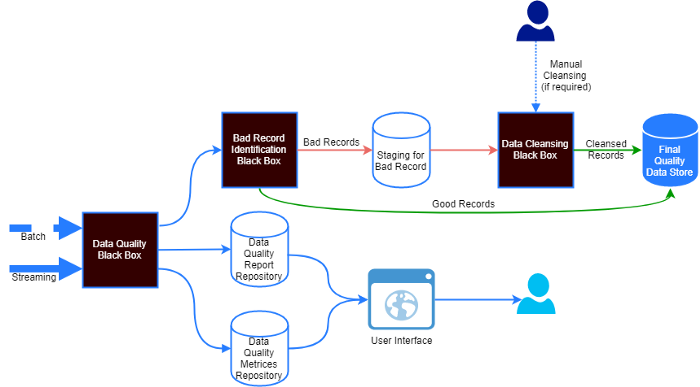# Data Validation at Scale with Spark/DatabricksA typical data ingestion pipeline with data quality
• Missing values that can lead to failures in production system that require non-null values (NullPointerException)?
• How changes in the distribution of data can lead to unexpected outputs of machine learning models?
• How aggregations of incorrect data can lead to wrong business decisions?
1. hasSize — calculates the data frame size and runs the assertion on it.
2. isComplete — asserts on a column completion.
3. hasCompleteness — asserts on a column completion.
4. isUnique — asserts on a column uniqueness.
5. isPrimaryKey — asserts on a column(s) primary key characteristics.
6. hasUniqueness — asserts on uniqueness in a single or combined set of key columns.
7. hasDistinctness — distinctness in a single or combined set of key columns.
8. hasUniqueValueRatio — the unique value ratio in a single or combined set of key columns.
9. hasNumberOfDistinctValues — asserts on the number of distinct values a column has.
10. hasHistogramValues — asserts on column’s value distribution.
11. hasEntropy — asserts on a column entropy.
12. hasMutualInformation — asserts on a mutual information between two columns.
13. hasApproxQuantile — asserts on an approximated quantile.
14. hasMinLength — asserts on the minimum length of the column.
15. hasMaxLength — asserts on the maximum length of the column.
16. hasMin — asserts on the minimum of the column.
17. hasMax — asserts on the maximum of the column.
18. hasMean — asserts on the mean of the column.
19. hasSum — asserts on the sum of the column.
20. hasStandardDeviation — asserts on the standard deviation of the column.
21. hasApproxCountDistinct — asserts on the approximate count distinct of the given column.
22. hasCorrelation — asserts on the Pearson correlation between two columns.
23. satisfies — runs the given condition on the data frame.
24. hasPattern — checks for pattern compliance.
25. containsCreditCardNumber — verifies against a Credit Card pattern.
26. containsEmail — verifies against an e-mail pattern.
27. containsURL — verifies against an URL pattern.
28. containsSocialSecurityNumber — verifies against the Social security number pattern for the US.
29. hasDataType — verifies against the fraction of rows that conform to the given data type.
30. isNonNegative — asserts that a column contains no negative values.
31. isPositive — asserts that a column contains no negative values.
32. isLessThan — asserts that, in each row, the value of columnA < the value of columnB.
33. isLessThanOrEqualTo — asserts that, in each row, the value of columnA ≤ the value of columnB.
34. isGreaterThan — asserts that, in each row, the value of columnA > the value of columnB.
35. isGreaterThanOrEqualTo — asserts that, in each row, the value of columnA ≥ to the value of columnB.
36. isContainedIn — asserts that every non-null value in a column is contained in a set of predefined values.

--

--